Name:    Negative Exponents

Multiple Choice
Identify the choice that best completes the statement or answers the question.

1.

Simplify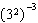.
 a.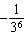b.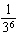c. -36 d. 36

2.

Which one of the following expressions does not equal 4?
 a.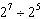c.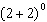b.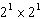d.3.

Which is the greatest number?
 a.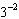c.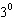b.d.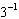4.

Evaluate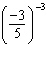.
 a.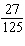c.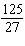b.d.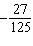5.

Determine the value of x so that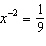.
 a. 18 c. 4.5 b. 9 d. 3